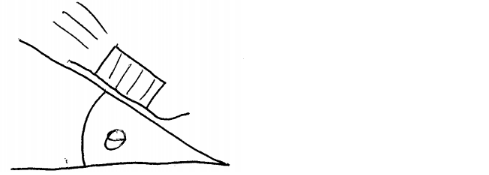# Solution: A box on a sled slides down a long, snow-overed slope. The hill slopes at a constant angle θ. There is a small coefficient of kinetic friction of 0.16. Find an expression for the acceleration.

###### Problem

A box on a sled slides down a long, snow-overed slope. The hill slopes at a constant angle θ. There is a small coefficient of kinetic friction of 0.16. Find an expression for the acceleration.